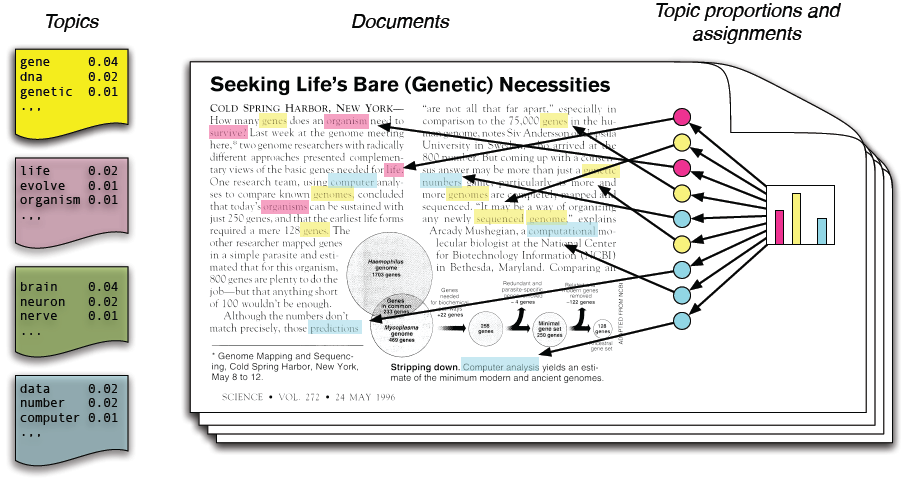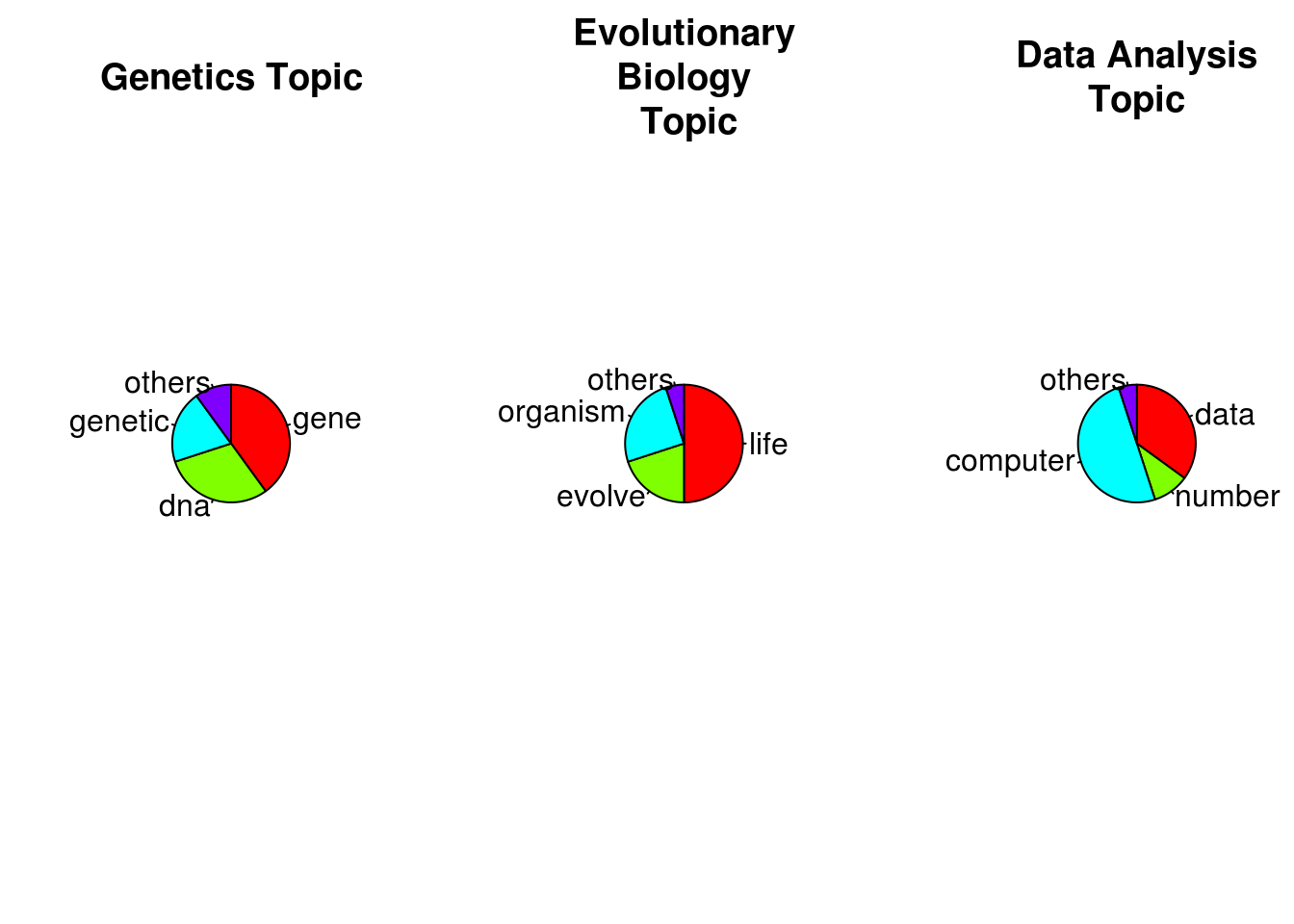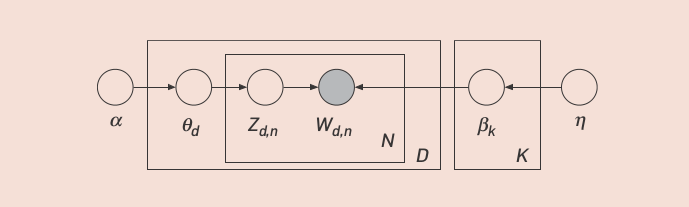In machine learning and natural language processing, a topic model is a type of statistical model for discovering the abstract “topics” that occur in a collection of documents.

# Latent Dirichlet Allocation

## IntuitionThe intuition behind LDA is that documents exhibit multiple topics.

For example, consider the article in Figure 1. This article, entitled “Seeking Life’s Bare (Genetic) Necessities,” is about using data analysis to determine the number of genes an organism needs to survive (in an evolutionary sense)

• By hand, we have highlighted different words that are used in the article.
• Words about data analysis, such as “computer” and “prediction,” are highlighted in blue;
• words about evolutionary biology, such as “life” and “organism,” are highlighted in pink;
• words about genetics, such as “sequenced” and “genes,” are highlighted in yellow.
• If we took the time to highlight every word in the article, you would see that this article blends genetics, data analysis, and evolutionary biology in different proportions.
• We exclude words, such as “and” “but” or “if,” which contain little topical content.

LDA is a statistical model of document collections that tries to capture this intuition. It is most easily described by its generative process, the imaginary random process by which the model assumes the documents arose. (The interpretation of LDA as a probabilistic model is fleshed out later.)

## Application

Although topic models were first described and implemented in the context of natural language processing, they have applications in other fields such as bioinformatics.

• Exploratory Analysis
• Discovery
• Browsing

## Definition

### Topic

We formally define a topic to be a distribution over a fixed vocabulary.

For example, the genetics topic has words about genetics with high probability and the evolutionary biology topic has words about evolutionary biology with high probability.

We assume that these topics are specified before any data has been generated.

library(ggplot2)
genetic.words <- c('gene', 'dna', 'genetic', 'others')
genetic.proportions <- c(40, 30, 20, 10)
par(fig=c(0, 0.3, 0, 1), new=TRUE)
pie(genetic.proportions, labels=genetic.words, main="Genetics Topic", clockwise=TRUE, col=rainbow(length(genetic.words)))
evolution.words <- c('life', 'evolve', 'organism', 'others')
evolution.proportions <- c(50, 20, 25, 5)
par(fig=c(0.35, 0.65, 0, 1), new=TRUE)
pie(evolution.proportions, labels=evolution.words, main="Evolutionary\nBiology\n Topic", clockwise=TRUE, col=rainbow(length(evolution.words)))
computing.words <- c('data', 'number', 'computer', 'others')
computing.proportions <- c(35, 10, 50, 5)
par(fig=c(0.7, 1, 0, 1), new=TRUE)
pie(computing.proportions, labels=computing.words, main="Data Analysis\nTopic", clockwise=TRUE, col=rainbow(length(computing.words)))### Generation Process (a.k.a model)

Now for each document in the collection, we generate the words in a two-stage process.

1. Randomly choose a distribution over topics.
2. For each word in the document
1. Randomly choose a topic from the distribution over topics in step #1.
2. Randomly choose a word from the corresponding distribution over the vocabulary.

This statistical model reflects the intuition that documents exhibit multiple topics.

Each document exhibits the topics in different proportion (step #1); each word in each document is drawn from one of the topics (step #2b), where the selected topic is chosen from the per-document distribution over topics (step #2a).

Example: In the example article, the distribution over topics would place probability on genetics, data analysis, and evolutionary biology, and each word is drawn from one of those three topics.

Notice that the next article in the collection might be about data analysis and neuroscience; its distribution over topics would place probability on those two topics.

This is the distinguishing characteristic of latent Dirichlet allocation all the documents in the collection share the same set of topics, but each document exhibits those topics in different proportion.

### Hidden Structure

As we described in the introduction, the goal of topic modeling is to automatically discover the topics from a collection of documents. The documents themselves are observed, while the topic structure; the topics, per-document topic distributions, and the per-document per-word topic; assignments; is hidden structure.

The central computational problem for topic modeling is to use the observed documents to infer the hidden topic structure. This can be thought of as; reversing; the generative process; what is the hidden structure that likely generated the observed collection?

## Probabilistic Modeling

LDA and other topic models are part of the larger field of probabilistic modeling.

In generative probabilistic modeling,

• we treat our data as arising from a generative process that includes hidden variables.
• This generative process defines a joint probability distribution over both the observed and hidden random variables.
• We perform data analysis by using that joint distribution to compute the conditional distribution of the hidden variables given the observed variables. * This conditional distribution is also called the posterior distribution.

## Describe formallyWe can describe LDA more formally with the following notation.

• The topics are $$b_{1:K}$$ , where each $$b_k$$ is a distribution over the vocabulary (the distributions over words at left in Figure 1).
• The topic proportions for the $$d^{th}$$ document are $$\theta_{d}$$ , where $$\theta_{d,k}$$ is the topic proportion for topic $$k$$ in document $$d$$ (the car- toon histogram in Figure 1).
• The topic assignments for the $$d^{th}$$ document are $$z_d$$ , where $$z_{d,n}$$ is the topic assignment for the $$n^{th}$$ word in document $$d$$ (the colored coin in Figure 1).
• Finally, the observed words for document d are $$w_d$$ ,where $$w_{d,n}$$ is the $$n^{th}$$ word in document d, which is an element from the fixed vocabulary.

## Step By Step

Keyword you must understand word, topic, document

Step 1: Generate word distributions for each topic

Step 2: Topic Distribution for document

Step 3: For each word, choose a topic and a word from that topic

## Inference

All you know is observed words

Intractable Problem

Gibbs Sampling, Variational Method

## More

• About intuition Intuitively, given that a document is about a particular topic, one would expect particular words to appear in the document more or less frequently: “dog” and “bone” will appear more often in documents about dogs, “cat” and “meow” will appear in documents about cats, and “the” and “is” will appear equally in both. A document typically concerns multiple topics in different proportions; thus, in a document that is 10% about cats and 90% about dogs, there would probably be about 9 times more dog words than cat words. A topic model captures this intuition in a mathematical framework, which allows examining a set of documents and discovering, based on the statistics of the words in each, what the topics might be and what each document’s balance of topics is.